Published

# Reed Switch Bike Speedometer using Particle Argons

This project will have bi-directional communication between two Particle Argons. It will calculate the speed of a bike and graph it.

BeginnerShowcase (no instructions)111## Things used in this project

### Hardware components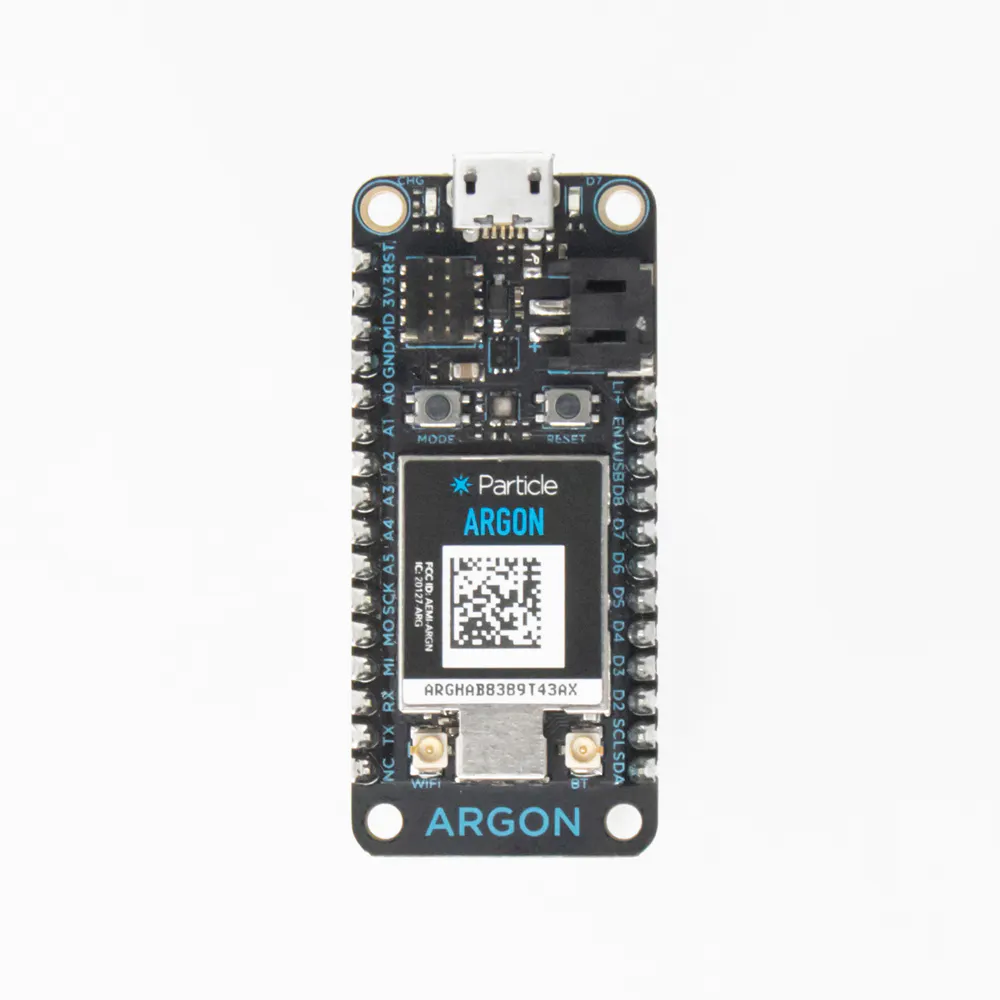Particle Argon
×2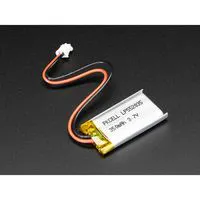Battery, 3.7 V
×1Reed Switch, Wide Gap
×1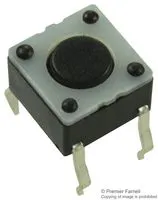Tactile Switch, Top Actuated
×1×2

### Software apps and online servicesParticle Build Web IDEThingSpeak API

## Schematics

### Argon on Bike

This is the Diagram for the Reed Switch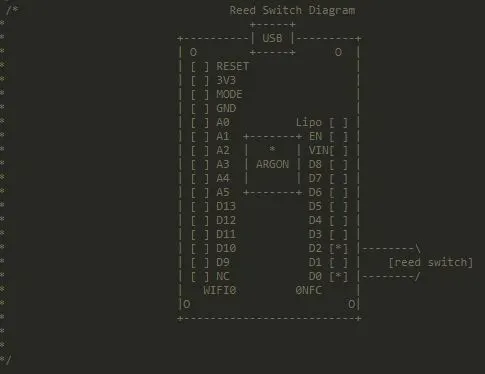### Stationary Argon

This is the diagram for the button.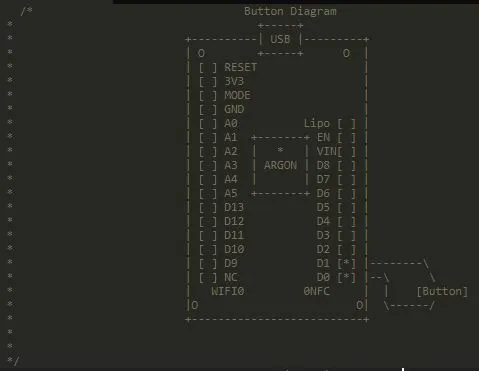## Code

### Argon on Bike

C/C++
```#include <math.h>;

int reedSwitch = D2; //Declaring pin for reed switch to be used. Pin D2 must be used for the reed switch on the particle
double radius = 12.5;   //hard coding in the radius of the wheel in inches for future conversion into circumference
double circumference;   //declaring the circumference variable
int totaltime;       //declaring the total time until the next trip on the reed switch variable
double Speed;           //declaring the Speed variable
int timer;           //declaring the timer to calculate the total time variable
int groundpin = D0;  // aux ground out.
const pin_t MY_LED = D7; //Use D7 LED
//const String key = "ME8J3G47QWK87YEP";

void myHandler(const char *event, const char *data){
//print passed Rssi value to realterm (for testing purpose)
Serial.println(event);
Serial.print("(From Argon2): ");
Serial.println(data);
//will Flash D7 LED to let know Speed was Updated
digitalWrite(MY_LED, HIGH);
delay(1s);
digitalWrite(MY_LED, LOW);

}

void StartTimer()
{
timer++;                        // Begin the timer and start counting
delayMicroseconds(9600);        // Delay to start after a 0.0096 seconds
}

void setup(){

//set up D7 led
pinMode(MY_LED, OUTPUT);

pinMode(groundpin, OUTPUT);                             //set up for reed witch ground

Serial.begin(9600);                                         // Start serial monitor after a few seconds. Mainly for testing code to get it to work.
attachInterrupt(D2, StartTimer, RISING);                    //Begins the count of seconds when the reed switch in pin D2 is set off
pinMode(reedSwitch, INPUT_PULLUP);                         // Sets the reed switch to be an input
timer = 0;                                                 // Sets timer to 0 seconds
//Speed = 0;                                                 // sets the speed to 0 seconds
totaltime = 0;                                             // sets the total time to start off at 0
}

void loop() {
if (millis() - totaltime >= 1000) {  // Gets varibales to go into the loop everytime the millis() - the total time elapsed is less than 1ms
detachInterrupt(D2);     // Stops the timer from the reading from pin D2 aka the reed switch

Speed = ((8.181818182*circumference)/(millis() - totaltime)*timer); // Calculates the speed of the bike. The 8.18 number is converting the time in seconds into hours and inches into feet.
timer = 0;               // Makes the timer go back to zero
totaltime = millis();        // Makes the total time the new time
attachInterrupt(D2, StartTimer, RISING);  // Make the StartTimer loop attach back

delay(30000);
Particle.publish("Speed", String(Speed), PRIVATE);

// return(Speed);//return speed value
}
//Realterm output (for testing purpose)
Serial.print("Speed(from reedSwitch): ");
Serial.println(Speed);
}
```

### Stationary Argon

C/C++
```int button = D1;     //input button is connected to D1 and the other terminal to ground.
int groundpin = D0;  // aux ground out.
int switchstate = 0; //a variable that will hold the current switch state
int lastswitchstate = 0; // a variable that will hold the previous switch state
const pin_t MY_LED = D7;
String Speed;        //declare speed value.
char publishString;
//volatile bool mailsend = FALSE; //not needed

//Thingspeak key
const String key = "ME8J3G47QWK87YEP";

// fuction for subcribe, this will update speed
void myHandler(const char *event, const char *data){
//print passed speed value to realterm (for testing purpose)
Serial.println(event);
Serial.print("(from Argon1): ");
Serial.println(data);
//sets subscribed Speed sata to local as Speed variable
Speed=data;
//will Flash D7 LED to let know Speed was Updated
digitalWrite(MY_LED, HIGH);
delay(1s);
digitalWrite(MY_LED, LOW);

}

void setup(){
//set up D7 led
pinMode(MY_LED, OUTPUT);
//update Speed value
Particle.subscribe("Speed", myHandler, MY_DEVICES);

Serial.begin(9600);
//set up for button
pinMode(button, INPUT_PULLUP);
pinMode(groundpin, OUTPUT);

rssisend = TRUE; //for web hook
}

void loop() {

lastswitchstate = switchstate;  //copy the previous switch state to the last switch state
if (lastswitchstate == 0 && (switchstate == 1)) { //if both are true then...  This is a bit more advanced than just detecting a input state. It detects an edge of a signal to prevent overloading the cloud.
//"==" compares the two values.  If equal it takes a value of "true" or 1.
// Particle.publish("data-start/stop", String(Speed), PRIVATE);  //send the event to the particle cloud
// delay(2000);

// Particle.subscribe("data-start/stop", string(Speed), PRIVATE);

// Serial.print();

}
}

//delay (2000);
static int count = 0;
Serial.println(count++);

//sprintf(publishString,"%d",strength);

Particle.publish("thingSpeakWrite_All", +
"{ \"1\": \"" + String(rssival) + "\"," +
"\"2\": \"" + String(Speed) + "\"," +
"\"3\": \"" + String(Speed) + "\"," +
"\"5\": \"" + String(Speed) + "\"," +  //channel 5 due to a legacy SOC channel being calculated on thingspeak via a react.
//"\"5\": \"" + String(var4) + "\"," +
"\"k\": \"" + key + "\" }", 60, PRIVATE, WITH_ACK);

if (success == TRUE) {
//delay(10000);
rssisend = FALSE;                        //if sent, then turn of the send flag, otherwise let it try again.
}
}
```

### Untitled file

C/C++
`No preview (download only).`

### Untitled file

C/C++
`No preview (download only).`

## Credits

### Matthew Love

1 project • 1 follower

### Brett Sackeli

0 projects • 0 followers